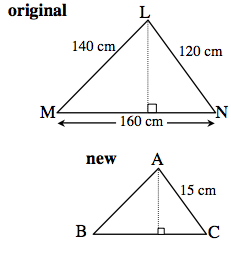### Home > MC2 > Chapter 9 > Lesson 9.3.3 > Problem9-113

9-113.
1. One of the triangles below is a reduction of the other. Find each of the missing parts listed below. Homework Help ✎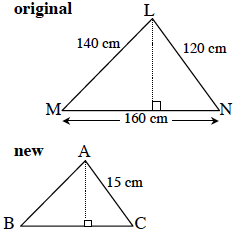1. Find the length of side BC.

2. Find the length of side AB.

3. Find the ratio of the areas of the two triangles.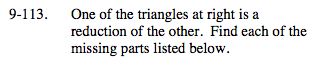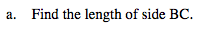What is the scale factor between the two triangles?

$\frac{120}{15} = \frac{160}{x}$

x = 20 cm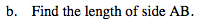See (a).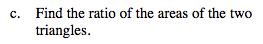If the ratio of the perimeters is the scale factor, the ratio of the areas is the scale factor squared.

$\frac{1}{64}$